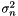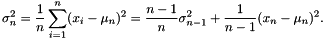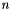#Boost C++ Libraries

...one of the most highly regarded and expertly designed C++ library projects in the world.

This is the documentation for an old version of Boost. Click here to view this page for the latest version.

## Struct template variance_impl

boost::accumulators::impl::variance_impl — Iterative calculation of variance.

## Synopsis

```// In header: <boost/accumulators/statistics/variance.hpp>

template<typename Sample, typename MeanFeature, typename Tag>
struct variance_impl {
// types
typedef numeric::functional::fdiv< Sample, std::size_t >::result_type result_type;

// construct/copy/destruct
template<typename Args> variance_impl(Args const &);

// public member functions
template<typename Args> void operator()(Args const &);
result_type result(dont_care) const;
};```

## Description

Iterative calculation of sample varianceaccording to the formula

Equation 1.31.where

Equation 1.32.is the estimate of the sample mean andis the number of samples.

Note that the sample variance is not defined for.

A simplification can be obtained by the approximate recursion

Equation 1.33.because the difference

Equation 1.34.converges to zero as. However, for smallthe difference can be non-negligible.

### `variance_impl` public construct/copy/destruct

1. `template<typename Args> variance_impl(Args const & args);`

### `variance_impl` public member functions

1. `template<typename Args> void operator()(Args const & args);`
2. `result_type result(dont_care) const;`
 Copyright © 2005, 2006 Eric Niebler Distributed under the Boost Software License, Version 1.0. (See accompanying file LICENSE_1_0.txt or copy at http://www.boost.org/LICENSE_1_0.txt)